Updating search results...

# 58 Results

View
Selected filters:
• Slope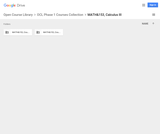Unrestricted Use
CC BY
Rating
0.0 stars

This contemporary calculus course is the third in a three-part sequence. In this course students continue to explore the concepts, applications, and techniques of Calculus - the mathematics of change. Calculus has wide-spread application in science, economics and engineering, and is a foundation college course for further work in these areas. This is a required class for most science and mathematics majors.Login: guest_oclPassword: ocl

Subject:
Calculus
Material Type:
Full Course
Homework/Assignment
Lecture Notes
Lesson Plan
Reading
Syllabus
Provider:
Washington State Board for Community & Technical Colleges
Provider Set:
Open Course Library
Date Added:
10/31/2011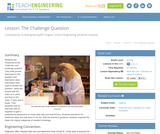Read the Fine Print
Educational Use
Rating
0.0 stars

Students are introduced to the "Walk the Line" challenge question. They write journal responses to the question and brainstorm what information they need to answer the question. Ideas are shared with the class (or in pairs and then to the class, if class size is large). Then students read an interview with an engineer to gain a professional perspective on linear data sets and best-fit lines. Students brainstorm for additional ideas and add them to the list. With the teacher's guidance, students organize the ideas into logical categories of needed knowledge.

Subject:
Engineering
Mathematics
Material Type:
Lesson Plan
Provider:
TeachEngineering
Provider Set:
TeachEngineering
Author:
Aubrey Mckelvey
Date Added:
09/18/2014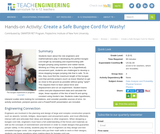Read the Fine Print
Educational Use
Rating
0.0 stars

Students learn about the role engineers and mathematicians play in developing the perfect bungee cord length by simulating and experimenting with bungee jumping using washers and rubber bands. Working as if they are engineers for a (hypothetical) amusement park, students are challenged to develop a show-stopping bungee jumping ride that is safe. To do this, they must find the maximum length of the bungee cord that permits jumpers (such as brave Washy!) to get as close to the ground as possible without going "splat"! This requires them to learn about force and displacement and run an experiment. Student teams collect and plot displacement data and calculate the slope, linear equation of the line of best fit and spring constant using Hooke's law. Students make hypotheses, interpret scatter plots looking for correlations, and consider possible sources of error. An activity worksheet, pre/post quizzes and a PowerPoint® presentation are included.

Subject:
Mathematics
Statistics and Probability
Physical Science
Physics
Material Type:
Activity/Lab
Provider:
TeachEngineering
Provider Set:
TeachEngineering
Author:
Marc Frank
Date Added:
02/17/2017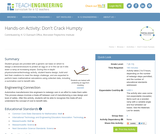Read the Fine Print
Educational Use
Rating
0.0 stars

Student groups are provided with a generic car base on which to design a device/enclosure to protect an egg on or in the car as it rolls down a ramp at increasing slopes. During this in-depth physics/science/technology activity, student teams design, build and test their creations to meet the design challenge, and are expected to perform basic mathematical calculations using collected data, including a summative cost to benefit ratio.

Subject:
Engineering
Physics
Material Type:
Activity/Lab
Provider:
TeachEngineering
Provider Set:
TeachEngineering
Author:
Justin Riley
Ryan St. Gelais
Scott Beaurivage
Date Added:
09/18/2014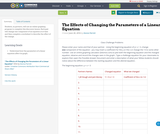Conditional Remix & Share Permitted
CC BY-NC-SA
Rating
2.0 stars

Students, in partners, will use an online graphing program to complete this discovery lesson. Each pair will change one component of an equation at at time and then complete a worksheet to describe the effect of the change.

Subject:
Mathematics
Material Type:
Activity/Lab
Author:
Donna Parrish
Date Added:
06/20/2016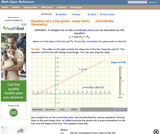Read the Fine Print
Rating
0.0 stars

An interactive applet and associated web page that demonstrate the equation of a line in point-slope form. The user can move a slider that controls the slope, and can drag the point that defines the line. The graph changes accordingly and equation for the line is continuously recalculated with every slider and / or point move. The grid, axis pointers and coordinates can be turned on and off. The equation display can be turned off to permit class exercises and then turned back on the verify the answers. The applet can be printed as it appears on the screen to make handouts. The web page has a full description of the concept of the equation of a line in point - slope form, a worked example and has links to other pages relating to coordinate geometry. Applet can be enlarged to full screen size for use with a classroom projector. This resource is a component of the Math Open Reference Interactive Geometry textbook project at http://www.mathopenref.com.

Subject:
Geometry
Material Type:
Reading
Simulation
Provider:
Math Open Reference
Author:
John Page
Date Added:
02/16/2011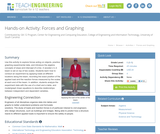Read the Fine Print
Educational Use
Rating
0.0 stars

Use this activity to explore forces acting on objects, practice graphing experimental data, and introduce the algebra concepts of slope and intercept of a line. A wooden 2 x 4 beam is set on top of two scales. Students learn how to conduct an experiment by applying loads at different locations along the beam, recording the exact position of the applied load and the reaction forces measured by the scales at each end of the beam. In addition, students analyze the experiment data with the use of a chart and a table, and model/graph linear equations to describe relationships between independent and dependent variables.

Subject:
Engineering
Mathematics
Physics
Material Type:
Activity/Lab
Provider:
TeachEngineering
Provider Set:
TeachEngineering
Author:
Ivanka Todorova
Jed Lyons
John Brader
Veronica Addison
Date Added:
09/18/2014
Remix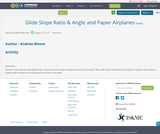Conditional Remix & Share Permitted
CC BY-NC-SA
Rating
0.0 stars

Students will calculate the glide slope ratio and angle of paper airplanes they have created. They will make claims about the type of airplane and support claims with evidence and reasoning from their class data.

Subject:
Algebra
Material Type:
Activity/Lab
Author:
STEMToolkit Administrator
Date Added:
04/29/2021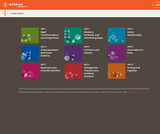Unrestricted Use
CC BY
Rating
0.0 stars

Student-facing 8th grade math resources. Covers transformations & congruence, slope, similarity, linear relationships, associations in data, volume, functions, scientific notation, the pythagorean theorem and irrational numbers.

Subject:
Mathematics
Material Type:
Unit of Study
Provider:
Open Up Resources
Date Added:
05/21/2019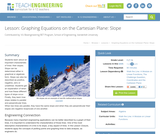Read the Fine Print
Educational Use
Rating
0.0 stars

Students learn about an important characteristic of lines: their slopes. Slope can be determined either in graphical or algebraic form. Slope can also be described as positive, negative, zero or undefined. Students get an explanation of when and how these different types of slope occur. Finally, they learn how slope relates to parallel and perpendicular lines. When two lines are parallel, they have the same slope and when they are perpendicular their slopes are negative reciprocals of one another.

Subject:
Engineering
Mathematics
Algebra
Material Type:
Lesson Plan
Provider:
TeachEngineering
Provider Set:
TeachEngineering
Author:
Aubrey Mckelvey
Date Added:
09/18/2014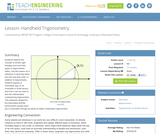Read the Fine Print
Educational Use
Rating
0.0 stars

Students explore the concept of similar right triangles and how they apply to trigonometric ratios. Use this lesson as a refresher of what trig ratios are and how they work. In addition to trigonometry, students explore a clinometer app on an Android® or iOS® device and how it can be used to test the mathematics underpinning trigonometry. This prepares student for the associated activity, during which groups each put a clinometer through its paces to better understand trigonometry.

Subject:
Engineering
Mathematics
Trigonometry
Material Type:
Lesson Plan
Provider:
TeachEngineering
Provider Set:
TeachEngineering
Author:
Scott Burns
Date Added:
09/18/2014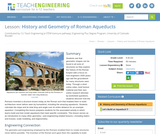Read the Fine Print
Educational Use
Rating
0.0 stars

Students see that geometric shapes can be found in all sorts of structures as they explore the history of the Roman Empire with a focus on how engineers 2000 years ago laid the groundwork for many structures seen today. Through a short online video, brief lecture material and their own online research directed by worksheet questions, students discover how the Romans invented a structure known today as the Roman arch that enabled them to build architecture never before seen by humankind, including the amazing aqueducts. Students calculate the slope and its total drop and angle over its entire distance for an example aqueduct. Completing this lesson prepares students for the associated activity in which teams build and test model aqueducts that meet specific constraints. This lesson serves as an introduction to many other geometry—and engineering-related lessons—including statics and trusses, scale modeling, and trigonometry.

Subject:
Mathematics
Geometry
Material Type:
Lesson
Provider:
TeachEngineering
Author:
Lauchlin Blue
Malinda Zarske
Nathan Coyle
Date Added:
02/07/2017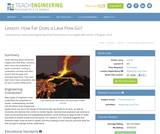Read the Fine Print
Educational Use
Rating
0.0 stars

While learning about volcanoes, magma and lava flows, students learn about the properties of liquid movement, coming to understand viscosity and other factors that increase and decrease liquid flow. They also learn about lava composition and its risk to human settlements.

Subject:
Engineering
Mathematics
Physics
Material Type:
Lesson Plan
Provider:
TeachEngineering
Provider Set:
TeachEngineering
Author:
Brittany Enzmann
Date Added:
09/18/2014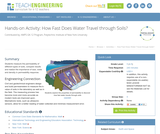Read the Fine Print
Educational Use
Rating
0.0 stars

Students measure the permeability of different types of soils, compare results and realize the importance of size, voids and density in permeability response.

Subject:
Engineering
Mathematics
Material Type:
Activity/Lab
Provider:
TeachEngineering
Provider Set:
TeachEngineering
Author:
Eduardo Suescun
Magued Iskander
Russ Holstein
Ryan Cain
Date Added:
09/18/2014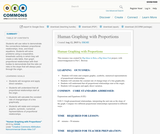Conditional Remix & Share Permitted
CC BY-NC-SA
Rating
0.0 stars

Students will use ratios to demonstrate the connections between proportional relationships, lines, and linear equations. Students will solve problems using a cooperative, kinesthetic activity in which they will create a ratio table, then graph proportional relationships with their bodies to demonstrate that the ratio (or rate) is the slope that will always pass through the origin.

Subject:
Mathematics
Material Type:
Activity/Lab
Teaching/Learning Strategy
Author:
Admin
Date Added:
01/28/2016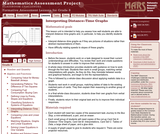Only Sharing Permitted
CC BY-NC-ND
Rating
4.0 stars

This lesson unit is intended to help teachers assess how well students are able to interpret distanceĐtime graphs and, in particular, to help you identify students who: interpret distanceĐtime graphs as if they are pictures of situations rather than abstract representations of them; and have difficulty relating speeds to slopes of these graphs.

Subject:
Mathematics
Material Type:
Assessment
Lesson Plan
Provider:
Shell Center for Mathematical Education
Provider Set:
Mathematics Assessment Project (MAP)
Date Added:
04/26/2013
Remix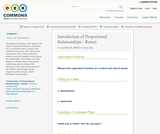Conditional Remix & Share Permitted
CC BY-NC-SA
Rating
0.0 stars

Throughout this lesson, the teacher will explain equivalent fractions, plot them on a coordinate plane, connect and extend those points, then discuss the importance of the relationship and explore what the students notice about the relationship. This lesson can help depend understanding of equivalent fractions as well as deepen the discussion into slope being similar to the fraction that we gave at the beginning of the lesson. (Math Solutions Training idea)

Subject:
Mathematics
Material Type:
Activity/Lab
Author:
Cortney Ray
Date Added:
08/08/2019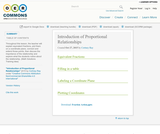Conditional Remix & Share Permitted
CC BY-NC-SA
Rating
0.0 stars

Throughout this lesson, the teacher will explain equivalent fractions, plot them on a coordinate plane, connect and extend those points, then discuss the importance of the relationship and explore what the students notice about the relationship. (Math Solutions Training idea)

Subject:
Mathematics
Material Type:
Activity/Lab
Author:
Cortney Ray
Date Added:
01/28/2016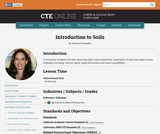Conditional Remix & Share Permitted
CC BY-NC-SA
Rating
0.0 stars

In this lesson students will learn about the basic soils components, importance of soils,main ideas in land evaluation including: texture, depth, slope and erosion and career possibilities.

Subject:
Agriculture
Material Type:
Lesson Plan
Provider:
Butte County Office of Education
Provider Set:
CTE Online
Author:
Jessica Fernandes
Date Added:
10/10/2016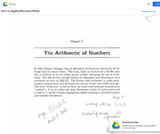Unrestricted Use
CC BY
Rating
0.0 stars

Introductory Algebra is the second of three classes in the developmental mathematics sequence. It provides a development of concepts of variables, expressions, and equations using symbolic algebra to represent primarily linear relationships both graphically and analytically. The concept of function will be developed for the application of linear equations and concepts of dependent and independent variable. Students will also learn to solve simultaneous linear equations as well as how to construct linear equations from slope and point information. Application problems will include geometric figure quantities, ratio and proportion, direct and indirect variation, and conversion of units. Finding the greatest common factor of a polynomial will also be included. The emphasis of the three semester sequence is fortification of mental calculation power with minimum reliance on digital calculation.

Subject:
Algebra
Material Type:
Full Course
Provider:
MassBay Community College
Author:
Ravindra Bhalla
Date Added:
05/13/2019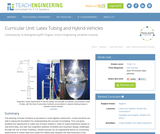Read the Fine Print
Educational Use
Rating
0.0 stars

The learning of linear functions is pervasive in most algebra classrooms. Linear functions are vital in laying the foundation for understanding the concept of modeling. This unit gives students the opportunity to make use of linear models in order to make predictions based on real-world data, and see how engineers address incredible and important design challenges through the use of linear modeling. Student groups act as engineering teams by conducting experiments to collect data and model the relationship between the wall thickness of the latex tubes and their corresponding strength under pressure (to the point of explosion). Students learn to graph variables with linear relationships and use collected data from their designed experiment to make important decisions regarding the feasibility of hydraulic systems in hybrid vehicles and the necessary tube size to make it viable.

Subject:
Engineering
Mathematics
Functions
Physics
Material Type:
Unit of Study
Provider:
TeachEngineering
Provider Set:
TeachEngineering
Author:
Carleigh Samson
Erik Bowen
Date Added:
09/18/2014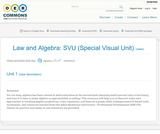Conditional Remix & Share Permitted
CC BY-NC-SA
Rating
0.0 stars

For too long, algebra has been viewed in adult education as the second most daunting math (second only to fractions), and now it is time to make algebra as approachable as adding. This resource will help you to discover some new approaches to teaching algebra as patterns, rules, equations, and lines on a graph while utilizing research-based tools, techniques, and resources learned from the Adult Numeracy Instruction – Professional Development (ANI-PD). Hands-on practice and ready-to-use resources are provided.

Subject:
Mathematics
Material Type:
Activity/Lab
Diagram/Illustration
Homework/Assignment
Teaching/Learning Strategy
Unit of Study
Author:
Heidi Schuler-Jones
Date Added:
01/28/2016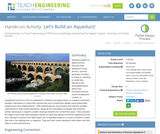Read the Fine Print
Educational Use
Rating
0.0 stars

Students explore in detail how the Romans built aqueducts using arches—and the geometry involved in doing so. Building on what they learned in the associated lesson about how innovative Roman arches enabled the creation of magnificent structures such as aqueducts, students use trigonometry to complete worksheet problem calculations to determine semicircular arch construction details using trapezoidal-shaped and cube-shaped blocks. Then student groups use hot glue and half-inch wooden cube blocks to build model aqueducts, doing all the calculations to design and build the arches necessary to support a water-carrying channel over a three-foot span. They calculate the slope of the small-sized aqueduct based on what was typical for Roman aqueducts at the time, aiming to construct the ideal slope over a specified distance in order to achieve a water flow that is not spilling over or stagnant. They test their model aqueducts with water and then reflect on their performance.

Subject:
Mathematics
Geometry
Material Type:
Activity/Lab
Provider:
TeachEngineering
Author:
Lauchlin Blue
Malinda Zarske
Nathan Coyle
Date Added:
02/07/2017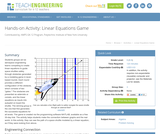Read the Fine Print
Educational Use
Rating
0.0 stars

Students groups act as aerospace engineering teams competing to create linear equations to guide space shuttles safely through obstacles generated by a modeling game in level-based rounds. Each round provides a different configuration of the obstacle, which consists of two "gates." The obstacles are presented as asteroids or comets, and the linear equations as inputs into autopilot on board the shuttle. The winning group is the one that first generates the successful equations for all levels. The game is created via the programming software MATLAB, available as a free 30-day trial. The activity helps students make the connection between graphs and the real world. In this activity, they can see the path of a space shuttle modeled by a linear equation, as if they were looking from above.

Subject:
Engineering
Astronomy
Material Type:
Activity/Lab
Provider:
TeachEngineering
Provider Set:
TeachEngineering
Author:
Stanislav Roslyakov
Date Added:
09/18/2014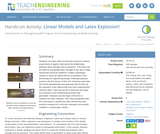Read the Fine Print
Educational Use
Rating
0.0 stars

Students use latex tubes and bicycle pumps to conduct experiments to gather data about the relationship between latex strength and air pressure. Then they use this data to extrapolate latex strength to the size of latex tubing that would be needed in modern passenger sedans to serve as hybrid vehicle accelerators, thus answering the engineering design challenge question posed in the first lesson of this unit. Students input data into Excel spreadsheets and generate best fit lines by the selection of two data points from their experimental research data. They discuss the y-intercept and slope as it pertains to the mathematical model they generated. Students use the slope of the line to interpret the data collected. Then they extrapolate with this information to predict the latex dimensions that would be required for a full-size hydraulic accumulator installed in a passenger vehicle.

Subject:
Career and Technical Education
Mathematics
Algebra
Material Type:
Activity/Lab
Provider:
TeachEngineering
Provider Set:
TeachEngineering
Author:
Carleigh Samson
Date Added:
02/17/2017Only Sharing Permitted
CC BY-NC-ND
Rating
5.0 stars

This lesson unit is intended to help teahcers assess how well students are able to interpret speed as the slope of a linear graph and translate between the equation of a line and its graphical representation.

Subject:
Algebra
Material Type:
Assessment
Lesson Plan
Provider:
Shell Center for Mathematical Education
Provider Set:
Mathematics Assessment Project (MAP)
Date Added:
04/26/2013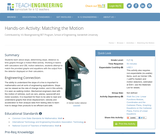Read the Fine Print
Educational Use
Rating
0.0 stars

Students learn about slope, determining slope, distance vs. time graphs through a motion-filled activity. Working in teams with calculators and CBL motion detectors, students attempt to match the provided graphs and equations with the output from the detector displayed on their calculators.

Subject:
Engineering
Mathematics
Physics
Material Type:
Activity/Lab
Provider:
TeachEngineering
Provider Set:
TeachEngineering
Author:
Aubrey McKelvey
Date Added:
09/18/2014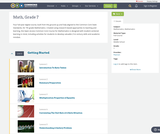Conditional Remix & Share Permitted
CC BY-NC
Rating
0.0 stars

Four full-year digital course, built from the ground up and fully-aligned to the Common Core State Standards, for 7th grade Mathematics. Created using research-based approaches to teaching and learning, the Open Access Common Core Course for Mathematics is designed with student-centered learning in mind, including activities for students to develop valuable 21st century skills and academic mindset.

Subject:
Mathematics
Material Type:
Full Course
Provider:
Pearson
Date Added:
10/06/2016Rating
0.0 stars

Proportional Relationships

Type of Unit: Concept

Prior Knowledge

Students should be able to:

Understand what a rate and ratio are.
Make a ratio table.
Make a graph using values from a ratio table.

Lesson Flow

Students start the unit by predicting what will happen in certain situations. They intuitively discover they can predict the situations that are proportional and might have a hard time predicting the ones that are not. In Lessons 2–4, students use the same three situations to explore proportional relationships. Two of the relationships are proportional and one is not. They look at these situations in tables, equations, and graphs. After Lesson 4, students realize a proportional relationship is represented on a graph as a straight line that passes through the origin. In Lesson 5, they look at straight lines that do not represent a proportional relationship. Lesson 6 focuses on the idea of how a proportion that they solved in sixth grade relates to a proportional relationship. They follow that by looking at rates expressed as fractions, finding the unit rate (the constant of proportionality), and then using the constant of proportionality to solve a problem. In Lesson 8, students fine-tune their definition of proportional relationship by looking at situations and determining if they represent proportional relationships and justifying their reasoning. They then apply what they have learned to a situation about flags and stars and extend that thinking to comparing two prices—examining the equations and the graphs. The Putting It Together lesson has them solve two problems and then critique other student work.

Gallery 1 provides students with additional proportional relationship problems.

The second part of the unit works with percents. First, percents are tied to proportional relationships, and then students examine percent situations as formulas, graphs, and tables. They then move to a new context—salary increase—and see the similarities with sales taxes. Next, students explore percent decrease, and then they analyze inaccurate statements involving percents, explaining why the statements are incorrect. Students end this sequence of lessons with a formative assessment that focuses on percent increase and percent decrease and ties it to decimals.

Students have ample opportunities to check, deepen, and apply their understanding of proportional relationships, including percents, with the selection of problems in Gallery 2.

Subject:
Mathematics
Ratios and Proportions
Material Type:
Unit of Study
Provider:
PearsonConditional Remix & Share Permitted
CC BY-NC
Rating
0.0 stars

Students analyze the graph of a proportional relationship in order to find the approximate constant of proportionality, to write the related formula, and to create a table of values that lie on the graph.Key ConceptsThe constant of proportionality determines the steepness of the straight-line graph that represents a proportional relationship. The steeper the line is, the greater the constant of proportionality.On the graph of a proportional relationship, the constant of proportionality is the constant ratio of y to x, or the slope of the line.A proportional relationship can be represented in different ways: a ratio table, a graph of a straight line through the origin, or an equation of the form y = kx, where k is the constant of proportionality.Goals and Learning ObjectivesIdentify the constant of proportionality from a graph that represents a proportional relationship.Write a formula for a graph that represents a proportional relationship.Make a table for a graph that represents a proportional relationship.Relate the constant of proportionality to the steepness of a graph that represents a proportional relationship (i.e., the steeper the line is, the greater the constant of proportionality).

Subject:
Ratios and Proportions
Material Type:
Lesson Plan
Provider:
Pearson
Date Added:
09/21/2015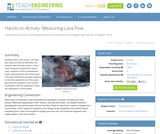Read the Fine Print
Educational Use
Rating
0.0 stars

Students learn how volume, viscosity and slope are factors that affect the surface area that lava covers. Using clear transparency grids and liquid soap, students conduct experiments, make measurements and collect data. They also brainstorm possible solutions to lava flow problems as if they were geochemical engineers, and come to understand how the properties of lava are applicable to other liquids.

Subject:
Engineering
Mathematics
Physics
Material Type:
Activity/Lab
Provider:
TeachEngineering
Provider Set:
TeachEngineering
Author:
Brittany Enzmann
Date Added:
09/18/2014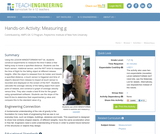Read the Fine Print
Educational Use
Rating
0.0 stars

Using the LEGO MINDSTORMS(TM) NXT kit, students construct experiments to measure the time it takes a free falling body to travel a specified distance. Students use the touch sensor, rotational sensor, and the NXT brick to measure the time of flight for the falling object at different release heights. After the object is released from its holder and travels a specified distance, a touch sensor is triggered and time of object's descent from release to impact at touch sensor is recorded and displayed on the screen of the NXT. Students calculate the average velocity of the falling object from each point of release, and construct a graph of average velocity versus time. They also create a best fit line for the graph using spreadsheet software. Students use the slope of the best fit line to determine their experimental g value and compare this to the standard value of g.

Subject:
Engineering
Physics
Material Type:
Activity/Lab
Provider:
TeachEngineering
Provider Set:
TeachEngineering
Author:
Jennifer Haghpanah
Keeshan Williams
Nicole Abaid
Date Added:
09/18/2014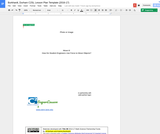Conditional Remix & Share Permitted
CC BY-NC
Rating
0.0 stars

Student engineers learn to understand force and motion and how it is effected by slope, design and weight carried.

Subject:
Physics
Material Type:
Activity/Lab
Provider:
Lane County STEM Hub
Provider Set:
Content in Context SuperLessons
Date Added:
06/26/2017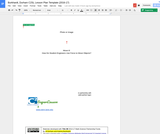Conditional Remix & Share Permitted
CC BY-NC
Rating
0.0 stars

The focus of this unit is to introduce the concepts of force and motion. Specifically this unit will address the forces of push, pull, gravity, and work. It also introduces students to the concepts of friction and slope. The unit begins with an introduction to the scientific method and addresses the differences between scientists and engineers. Students will be both scientists and engineers while completing this unit.

Subject:
Engineering
Physics
Material Type:
Activity/Lab
Provider:
Lane County STEM Hub
Provider Set:
Content in Context SuperLessons
Author:
Jill Durham
Katrina Burkhardt
Date Added:
06/26/2017Conditional Remix & Share Permitted
CC BY-NC-SA
Rating
0.0 stars

In this activity, learners work in groups to determine the mass and volume of four samples: glass marbles, steel washers or nuts, pieces of pine wood, and pieces of PVC pipe. Learners then plot the data points on a large class graph of mass vs. volume to discover that data points for a particular material form a straight line, the slope of which gives the density of the material.

Subject:
Physics
Material Type:
Activity/Lab
Provider:
Exploratorium
Author:
Don Rathjen
The Exploratorium
Date Added:
11/07/2009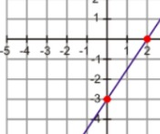Conditional Remix & Share Permitted
CC BY-NC
Rating
0.0 stars

This seminar will allow you to identify linear equations written in both point-slope and standard forms.  You will learn how to graph these equations as well as how to convert from one form to another.  You will discover why each form is useful in different ways in order to express different situations.StandardsCC.2.2.HS.D.10  Represent, solve, and interpret equations/inequalities and systems of equations/inequalities algebraically and graphically.

Subject:
Mathematics
Algebra
Material Type:
Lesson Plan
Author:
Bonnie Waltz
Tracy Rains
Deanna Mayers
Date Added:
10/15/2017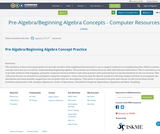Conditional Remix & Share Permitted
CC BY-NC-SA
Rating
4.0 stars

The intention of this curriculum guide is to provide teachers with supplemental materials to use to support students in strengthening their skills in various concept areas that are crucial for understanding beginning algebra. The activities are broken down by skill with links provided below. This is intended as a way to provide students with engaging, primarily computer-based activities to get extra practice with material that is covered elsewhere in the curriculum. This collection focuses on simulations and games using the computer—some resources may be ripe for teachers to develop unique activities to accompany the simulation and some possible suggestions are included with the descriptions. This series is intended to be pick-and-choose.

In this Curriculum Guide:

Activities and practice with: Integers, Exponents, Order of Operations, Distributive Property, Expressions, Equations and Basic Graphing

Subject:
Mathematics
Material Type:
Activity/Lab
Diagram/Illustration
Game
Interactive
Simulation
Author:
Lee Gargagliano
Date Added:
05/24/2018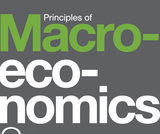Unrestricted Use
CC BY
Rating
0.0 stars

Principles of Macroeconomics 2e covers the scope and sequence of most introductory economics courses. The text includes many current examples, which are handled in a politically equitable way. The outcome is a balanced approach to the theory and application of economics concepts. The second edition has been thoroughly revised to increase clarity, update data and current event impacts, and incorporate the feedback from many reviewers and adopters.Changes made in Principles of Macroeconomics 2e are described in the preface and the transition guide to help instructors transition to the second edition.

Subject:
Social Science
Economics
Material Type:
Full Course
Provider:
Rice University
Provider Set:
OpenStax College
Date Added:
06/29/2017Unrestricted Use
CC BY
Rating
0.0 stars

In this Unit, you will learn about:How Individuals Make Choices Based on Their Budget ConstraintThe Production Possibilities Frontier and Social ChoicesConfronting Objections to the Economic Approach

Subject:
Applied Science
Economics
Material Type:
Module
Provider:
Rice University
Provider Set:
OpenStax College
Date Added:
09/20/2018